August 04, 2016

# Scanning Slits vs. Cameras

#### Which Type of Profiler

Our customers often ask us what type of beam profiler they should use to measure their laser beam(s). In this blog post, we explain the relative advantages and disadvantages of a scanning slit profiler and a camera-based profiler.

#### Scanning Slit Devices

In the early days of beam profiling, scanning slit or knife edge devices were the only beam profiling options available; however, with the advent of the CCD and CMOS sensors, camera detectors have become the laser beam profiler of choice. Scanning slit detectors are still used in a few applications where they have a technical advantage over camera based detectors. The scanning slit device is operated by moving material with a slit in it through a laser beam (see Fig. 1). A photodetector behind the material is then used to measure and record the summation of all the light entering the slit. If the speed at which the slit moves is known, then a profile of the beam along the slit’s axis of travel can be determined from the photodetector measurements (see Fig. 2a and Fig. 3a).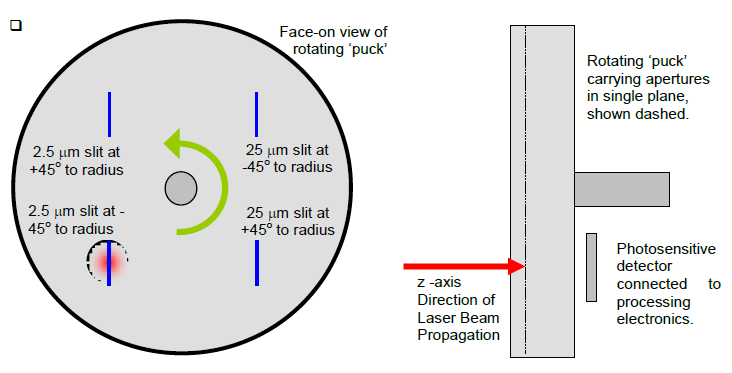Figure 1: Example of a scanning slit device. The puck shown (left) rotates the slits through the beams. Behind the puck (right) a photodetector records the summation of the energy entering the slit.

The first advantage a scanning slit has over a camera based detector is higher resolution. Currently, the smallest pixel size offered by laser profiling sensors is around 3 µm, so that the beam under measurement must be at least 30 µm (see our previous blog post on small beam width error). However, a scanning slit device delivers a significantly better resolution of 0.1 µm and allows beams down to 2 µm to be profiled. Thus, when measuring very small beams, a scanning slit device is preferred. In addition to profiling small beams, scanning slit devices have a large wavelength range. The silicon detectors in cameras have difficulty imaging wavelengths above 1200 nm, but scanning slit devices can use both silicon and InGaAs photodetectors. This significantly extends the wavelength range of the device and the DataRay Beam'R2 scanning slit detector can profile beams from 190–2500 nm.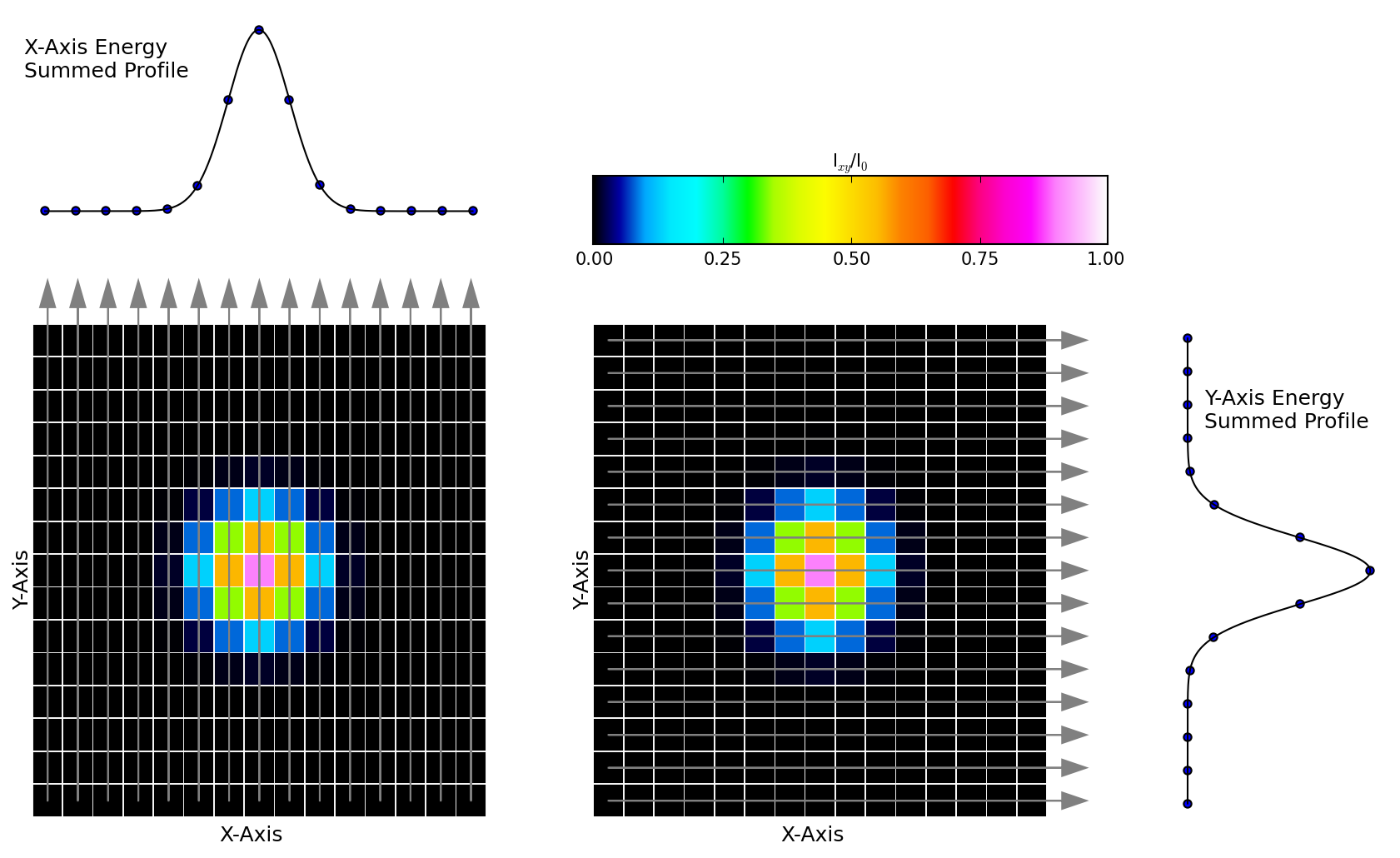(a)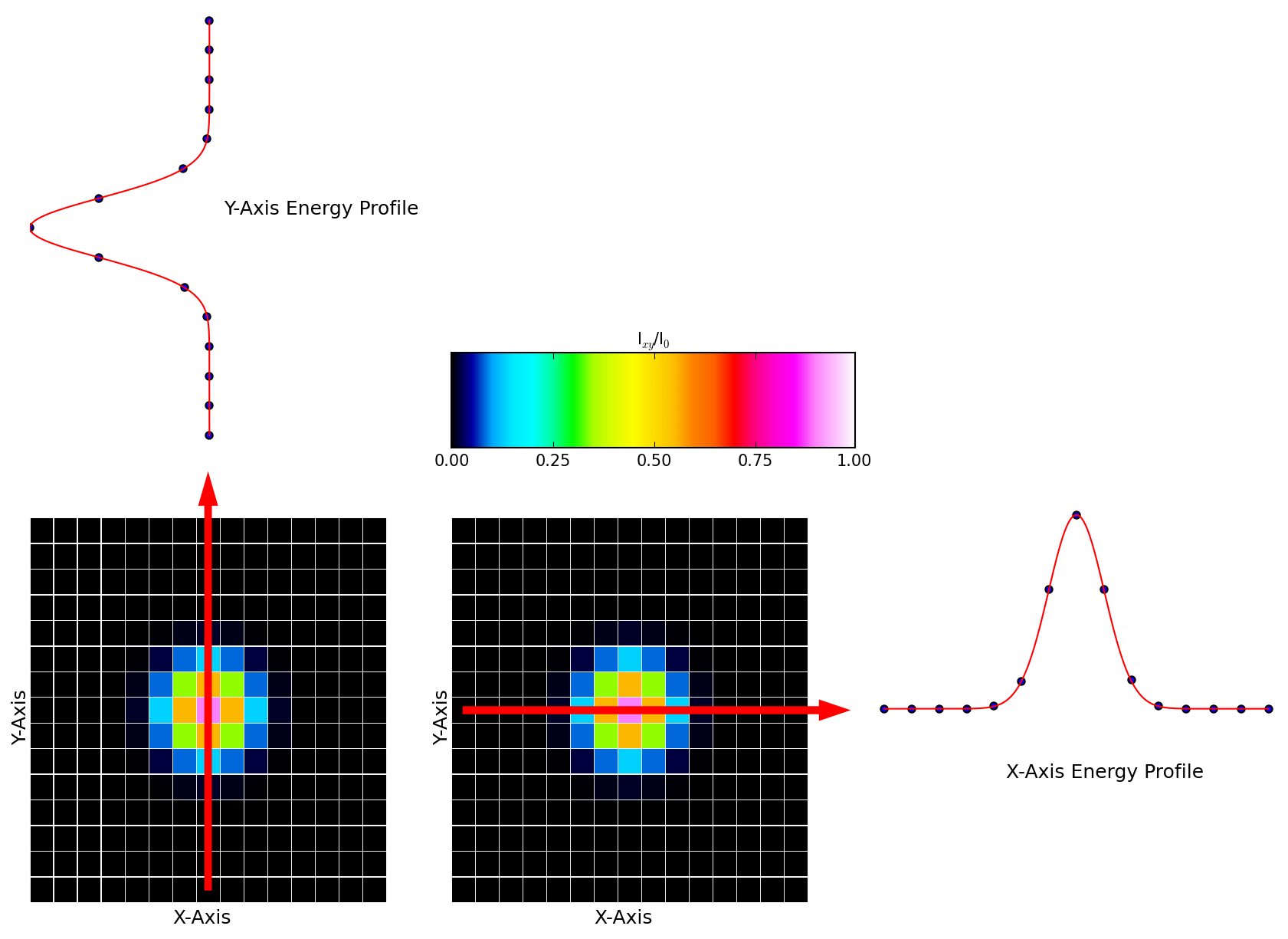(b)

Figure 2: (a) Example of a Gaussian beam undergoing measurement by a scanning slit device. The grey arrows denote the the portion of the beam’s energy that the slit allows through to the photodetector. The energy is measured by the photodetector and plotted as a point in the profile of the beam. The summed energy profiles are shown for both the x and y axes. (b) Example of a Gaussian beam undergoing measurement by a camera detector. The energy profiles are found by using a row (for the x-axis) or a column (for the y-axis) of pixels across the middle of the beam (denoted by the red arrows).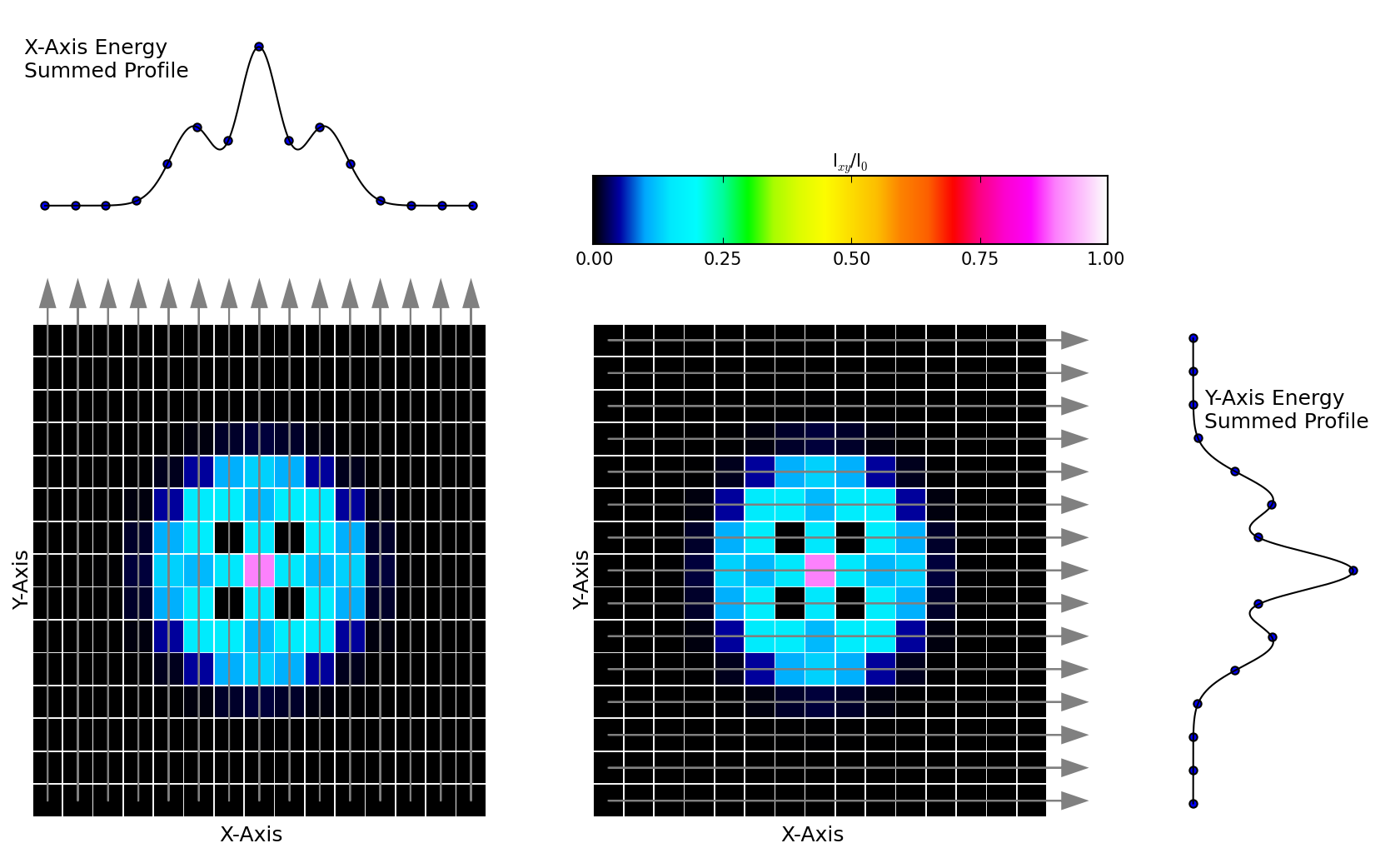(a)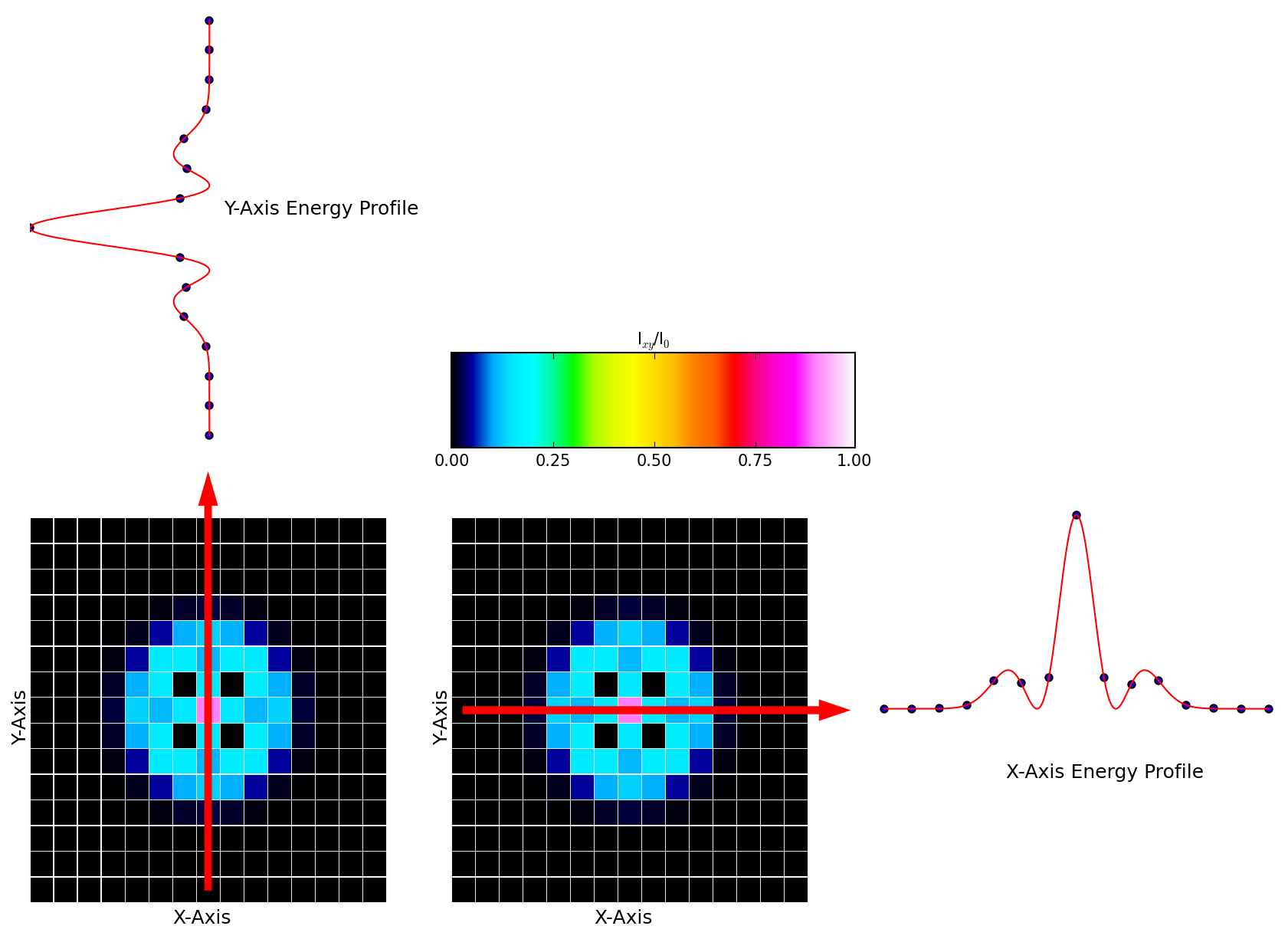(b)

Figure 3: (a) Example of a TEM$$_{10}$$ Laguerre-Gaussian beam undergoing measurement by a scanning slit device. The grey arrows denote the portion of the beam that is summed together to represent each point on the profile of the beam. (b) Example of a TEM$$_{10}$$ Laguerre-Gaussian beam undergoing measurement by a camera detector. The energy profiles are found by using a row (for the x-axis) or a column (for the y-axis) of pixels across the middle of the beam (denoted by the red arrows). Note that the summed profiles in (a) do not provide a direct representation of the actual beam profiles along the x and y axes in (b).

#### Cameras

Although cameras have a limited wavelength and a lower resolution than scanning slit devices, they show the full two dimensional profile of the beam, allowing more accurate beam measurements. A camera based detector determines the exact profile of the beam along the principal axes by using a row or column of pixels to measure the beam’s intensity distribution. Scanning slit devices show the summed beam profile along the axis of travel and for a relatively pure TEM$$_{00}$$ Gaussian beam this isn’t problematic; the summation along the slit is representative of the actual beam profile (see Fig. 2a and Fig. 2b). However, for higher-order modes, the summed profile of the beam doesn’t match the actual profile of the beam (see Fig. 3a and see Fig. 3b) so that the scanning slit returns an inaccurate beam profile.

Another advantage camera based detectors have over scanning slit devices is the ability to take more accurate beam measurements through use of the second moment. The second moment calculates the variance of the beam distribution to determine the beam width and other parameters. The equation for the beam width $$w_{x}$$ along one axis is given as

\begin{equation} w_{x}^2=2\frac{\displaystyle \iint (x-\bar{x})^2I(x, y) \,dx\,dy}{\displaystyle \iint I(x, y) \,dx\,dy} \label{sigma1} \end{equation}

where $$I(x, y)$$ is the beam’s intensity distribution across the sensor and $$\bar{x}$$ is the $$x$$ coordinate of the beam’s centroid. Although scanning slit detectors can use the second moment method, the calculations are done with only a one dimensional intensity distribution $$I(x)$$

\begin{equation} w_{x}^2=2\frac{\displaystyle \int (x-\bar{x})^2I(x) \,dx}{\displaystyle \int I(x) \,dx}\text{.} \label{sigma2} \end{equation}

The accuracy of the second moment measurement is higher when using a camera detector due to the use of the two dimensional data in the calculations.

#### Conclusion

Although scanning slit devices are advantageous when dealing with certain wavelength ranges or very small beams, camera based detectors are able to obtain a more complete profile of the beam. This is especially important when measuring beams with significant amounts of higher-order mode content. If you have any questions about which beam profiler is best for your application, please contact us. We have years of experience in laser profiling and look forward to working with you.

Posted by: Lucas Hofer